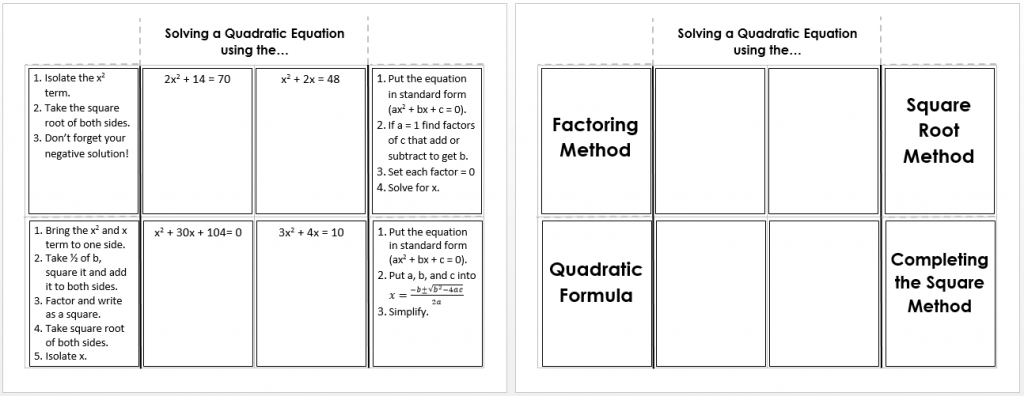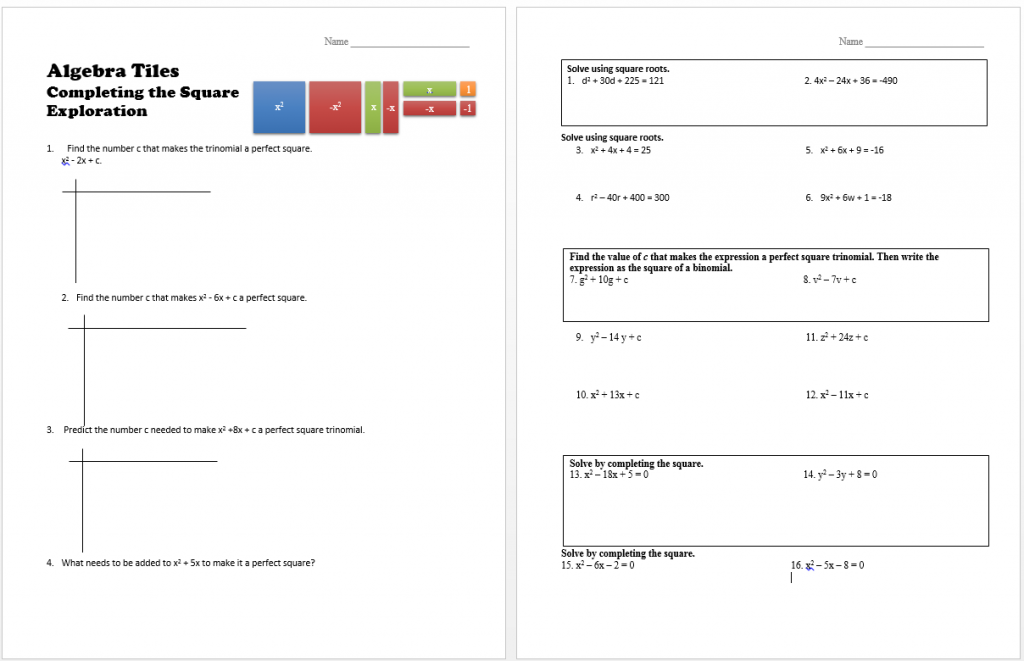/, Algebra Tiles, PreCalculus/Solving Quadratic Equations

There are many ways to solve quadratic equations. The resources below can help you remember key concepts, discover new methods, and fine tune your skills.

Solving by factoring and graphing is a worksheet that contains three types of equations – perfect square trinomials, difference of squares, and factoring trinomials to solve. It also has two solving by graphing problems.

The Solving Quadratic Equations Foldable is a one-stop shop for four ways to solve quadratics. Add it to your interactive notebook to remind yourself of the steps.## Solving by Completing the Square

You can discover the steps of creating a perfect square trinomial by using Algebra Tiles. The Algebra Tiles  Completing the square exploration and notes sheet can help you master this method of solving quadratics.By | 2017-11-13T22:02:00+00:00 September 25th, 2015|Algebra II, Algebra Tiles, PreCalculus|0 Comments

### About the Author: Stacie Bender